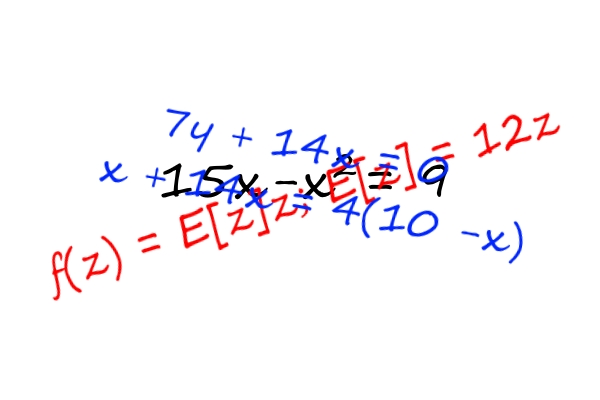### Back to algebra

Until we get to algebra, returning to algebra is a consistent theme in our daily practice until we take the step from solving algebra problems to using algebra to solve them.

Back to algebraTeaching algebra to a child who is not ready for algebra is like teaching arithmetic to a 2nd grader without letting the child memorize math facts. In the latter situation, the child builds number sense. In the former, the child is building algebra sense.

We started doing algebra problems about a year ago, and now can silently look at a problem that requires algebra and derive the equation not using algebra. All of the basic problem solving skills are required to get to this point, especially the ability to work diligently for an extended period in the face of frustration. The frustration in this case is me watching my child not use algebra. It's worth it.

Here is the problem for this article: Jack London wrote Moon Face in 68 days. If he would have written an extra page a day, it would have taken only 51 days. How many pages are in the book?

False start

A child steeped in problem solving might say 'n times 17 = 51'. Is this the correct answer? I don't know. I have to work it out, because I didn't have an academic coach with the forsight to not teach me algebra when algebra is so obviously needed.

I presume that the child split this problem into 2 sub problems on the way to 17n = 51 but can't untwist the jumble of thinking to explain how he did it.

Where genius is born

If you withhold prepackaged math concepts from your child like carry the one in double digit addition, long division, and in this case algebra, you're opening the door to advanced thinking. Once you provide pre-packaged solution methods, the child's brain will shut down while they follow the steps in order and reduce the complexity of advanced math to applying arithmetic steps.

The time is coming in their academic career when the child will be required to think. The whole purpose of an academic career is to get the child to the point of thinking. A child who applies formulas and mechanisms to solve problems year-after-year will get to the point where thinking is required and fall apart academically. It usually happens in pre-algebra, if you are lucky and there's time to rectify the problem, but may happen in high school where the only recourse left is a tutor or the school's psychologist.

Coaching genius

At some point the child will a) have the correct answer, b) have an incorrect answer, or c) be totally stuck.

What's the difference between coaching a genius and coaching a complete math moron? Nothing other than how many of these problems you've done. Every math moron is a genius waiting to happen. If you think you have a math genius, you're using problems that are too easy.

When it's time to check the answer, I'll work out the answer verbally and with step-by-step algebraic equations. I ask a question for each step like 'How many pages is in the book if Jack wrote for 68 days?' 68p = n. Write the equation to describe writing an extra page a day for 51 days (not me, I'll wait 30 minutes for this) which is 51(p+1) = n. Now what do we do?

We do about 3 of these problems a week. At some point last year, I explained that n =n, and the fundamental theorem of algebra means that you can't solve 1 equation with 2 variables (does it?) so 68p = 51(p+1).

If it takes 10 minutes to answer this problem, and I don't see a step-by-step solution, it will take 20 minutes to do it properly with algebra. I'm inching toward the point where I can ask for the child to write down the step-by-step equations without my assistance. We're not there yet. I think my older one is there, because he's studying algebra in math class, but I'm out of the picture so I can't say for sure.

Getting to algebra

How long will it take for my little student to stop working out problems mentally and start using algebra? When these problems are no longer challenging, he'll start using algebra. It's as if his brain is trying to eek out perfect comprehension before substituting mental effort with abstract equations.

The bonus

A child with number sense knows he's wrong when he gets 15 x .3 = 45. A child with algebra sense needs no formulas. He will eventually need algebraic equations, but topics like profit and probability are just known without help. He'll have a dozen or so internal algorithms that he created himself. Occasionally I see one of these in action and I'm in awe.

It is impossible to use this pedagogy in a classroom setting. If I were to use this method in the average American 6th grade, parents would watch their children get most problems wrong between September and May, and these kids would probably fail a pre-algebra test on May 31. Then by June 15, they would ace anything on the SAT. I would be fired in October, so it's a mute point.